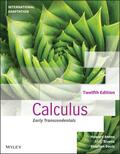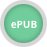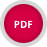John Wiley & Sons Calculus Early TranscendentalsCalculus: Early Transcendentals, 12th edition delivers a rigorous and intuitive exploration of calcu.. Product #: 978-1-119-82048-2 Regular price: \$61.59 \$61.59 Auf Lager

# Calculus Early Transcendentals12. Auflage Juni 2022
1232 Seiten, Softcover
Wiley & Sons Ltd

ISBN: 978-1-119-82048-2
John Wiley & Sons

Jetzt kaufen

### Preis: 65,90 €

Preis inkl. MwSt, zzgl. Versand

Weitere VersionenCalculus: Early Transcendentals, 12th edition delivers a rigorous and intuitive exploration of calculus, introducing polynomials, rational functions, exponentials, logarithms, and trigonometric functions early in the text. Using the Rule of Four, the authors present mathematical concepts from verbal, algebraic, visual, and numerical points of view. The book includes numerous exercises, applications, and examples that help readers learn and retain the concepts discussed within. This new adapted twelfth edition maintains those aspects of the previous editions that have led to the series success, at the same provides freshness to the new edition that would attract new users.

CHAPTER 1 Limits and Continuity

1.1 Limits (An Intuitive Approach)

1.2 Computing Limits

1.3 Limits at Infinity; End Behavior of a Function

1.4 Limits (Discussed More Rigorously)

1.5 Continuity

1.6 Trigonometric Functions

1.7 Inverse Trigonometric Functions

1.8 Exponential and Logarithmic Functions

CHAPTER 2 The Derivative

2.1 Tangent Lines and Rates of Change

2.2 The Derivative Function

2.3 Introduction to Techniques of Differentiation

2.4 The Product and Quotient Rules

2.5 Derivatives of Trigonometric Functions

2.6 The Chain Rule

CHAPTER 3 Differentiation

3.1 Implicit Differentiation

3.2 Derivatives of Logarithmic Functions

3.3 Derivatives of Exponential and Inverse Trigonometric Functions

3.4 Related Rates

3.5 Local Linear Approximation; Differentials

3.6 L'Hôpital's Rule; Indeterminate Forms

CHAPTER 4 The Derivative in Graphing and Applications

4.1 Analysis of Functions I: Increase, Decrease, and Concavity

4.2 Analysis of Functions II: Relative Extrema; Graphing Polynomials

4.3 Analysis of Functions III: Rational Functions, Cusps, and Vertical Tangents

4.4 Absolute Maxima and Minima

4.5 Applied Maximum and Minimum Problems

4.6 Rectilinear Motion

4.7 Newton's Method

4.8 Rolle's Theorem; Mean-Value Theorem

CHAPTER 5 Integration

5.1 An Overview of Area and Speed-Distance Problems

5.2 The Indefinite Integral

5.3 Integration by Substitution

5.4 The Definition of Area as a Limit; Sigma Notation

5.5 The Definite Integral

5.6 The Fundamental Theorem of Calculus

5.7 Rectilinear Motion Revisited Using Integration

5.8 Average Value of a Function and its Applications

5.9 Evaluating Definite Integrals by Substitution

5.10 Logarithmic and Other Functions Defined by Integrals

CHAPTER 6 Applications of the Definite Integral

6.1 Area Between Two Curves

6.2 Volumes by Slicing; Disks and Washers

6.3 Volumes by Cylindrical Shells

6.4 Length of a Plane Curve

6.5 Area of a Surface of Revolution

6.6 Work

6.7 Moments, Centers of Gravity, and Centroids

6.8 Fluid Pressure and Force

6.9 Hyperbolic Functions and Hanging Cables

CHAPTER 7 Principles of Integral Evaluation

7.1 An Overview of Integration Methods

7.2 Integration by Parts

7.3 Integrating Trigonometric Functions

7.4 Trigonometric Substitutions

7.5 Integrating Rational Functions by Partial Fractions

7.6Using Computer Algebra Systems and Tables of Integrals

7.7 Numerical Integration; Simpson's Rule

7.8 Improper Integrals

CHAPTER 8 Mathematical Modeling with Differential Equations

8.1 Modeling with Differential Equations

8.2 Separation of Variables

8.3 Slope Fields; Euler's Method

8.4 First-Order Differential Equations and Applications

8.5 Prey-Predator Model

CHAPTER 9 Parametric and Polar Curves; Conic Sections

9.1 Parametric Equations; Tangent Lines and Arc Length for Parametric Curves

9.2 Polar Coordinates

9.3 Tangent Lines, Arc Length, and Area for Polar Curves

9.4 Conic Sections

9.5 Rotation of Axes; Second-Degree Equations

9.6 Conic Sections in Polar Coordinates

CHAPTER 10 Sequence and Infinite Series

10.1 Sequences

10.2 Monotone Sequences

10.3 Infinite Series

10.4 Convergence Tests

10.5 The Comparison, Ratio, and Root Tests

10.6 Alternating Series; Absolute and Conditional Convergence

10.7 Maclaurin and Taylor Polynomials

10.8 Maclaurin and Taylor Series; Power Series

10.9 Convergence of Taylor Series

10.10 Differentiating and Integrating Power Series; Modeling with Taylor Series

CHAPTER 11 Three-dimensional Space; Vectors

11.1 Rectangular Coordinates in 3-space; Spheres; Cylindrical Surfaces

11.2 Vectors

11.3 Dot Product; Projections

11.4 Cross Product

11.5 Parametric Equations of Lines

11.6 Planes in 3-space

11.8 Cylindrical and Spherical Coordinates

CHAPTER 12 Vector-Valued Functions

12.1 Introduction to Vector-Valued Functions

12.2 Calculus of Vector-Valued Functions

12.3 Change of Parameter; Arc Length

12.4 Unit Tangent, Normal, and Binormal Vectors

12.5 Curvature

12.6 Motion Along a Curve

12.7 Kepler's Laws of Planetary Motion

CHAPTER 13 Partial Derivatives 13.1 Functions of Two or More Variables

13.2 Limits and Continuity

13.3 Partial Derivatives

13.4 Differentiability, Differentials, and Local Linearity

13.5 The Chain Rule

13.7 Tangent Planes and Normal Vectors

13.8 Maxima and Minima of Functions of Two Variables

13.9 Lagrange Multipliers

CHAPTER 14 Multiple Integrals

14.1 Double Integrals

14.2 Double Integrals Over Nonrectangular Regions

14.3 Double Integrals in Polar Coordinates

14.4 Surface Area; Parametric Surfaces

14.5 Triple Integrals

14.6 Triple Integrals in Cylindrical and Spherical Coordinates

14.7 Change of Variables in Multiple Integrals; Jacobians

14.8Centers of Gravity Using Multiple Integrals

CHAPTER 15 Vector Calculus

15.1 Vector Fields

15.2 Line Integrals

15.3 Independence of Path; Conservative Vector Fields

15.4 Green's Theorem

15.5 Surface Integrals

15.6 Applications of Surface Integrals; Flux

15.7 The Divergence Theorem

15.8 Stokes' Theorem

APPENDICES

A TRIGONOMETRY SUMMARY

B FUNCTIONS (SUMMARY)

C NEW FUNCTIONS FROM OLD (SUMMARY)

D FAMILIES OF FUNCTIONS (SUMMARY)

E Inverse Functions (Summary

INDEX Ind-1

WEB APPENDICES (online only)

A TRIGONOMETRY REVIEW

B FUNCTIONS

C NEW FUNCTIONS FROM OLD

D FAMILIES OF FUNCTIONS

E INVERSE FUNCTIONS

F REAL NUMBERS, INTERVALS, AND INEQUALITIES

G ABSOLUTE VALUE

H COORDINATE PLANES, LINES, AND LINEAR FUNCTIONS

I DISTANCE, CIRCLES, AND QUADRATIC EQUATIONS

J SOLVING POLYNOMIAL EQUATIONS

K GRAPHING FUNCTIONS USING CALCULATORS AND

COMPUTER ALGEBRA SYSTEMS

L SELECTED PROOFS

M EARLY PARAMETRIC EQUATIONS OPTION

N MATHEMATICAL MODELS

O THE DISCRIMINANT

P SECOND-ORDER LINEAR HOMOGENEOUS DIFFERENTIAL EQUATIONS

Chapter Web Projects: Expanding the Calculus Horizon (online only)